# RS Aggarwal Class 7 Solutions Chapter 3 Decimals Ex 3D

In this chapter, we provide RS Aggarwal Solutions for Class 7 Chapter 3 Decimals Ex 3D for English medium students, Which will very helpful for every student in their exams. Students can download the latest RS Aggarwal Solutions for Class 7 Chapter 3 Decimals Ex 3D Maths pdf, free RS Aggarwal Solutions Class 7 Chapter 3 Decimals Ex 3D Maths book pdf download. Now you will get step by step solution to each question.

### RS Aggarwal Solutions for Class 7 Chapter 3 Decimals Ex 3D Download PDF

Question 1.
Solution:
We know that a decimal divided by 10, the decimal point is shifted to the left by one place. Therefore
(i) 131.6 ÷ 10 = 13.16
(ii) 32.56 ÷ 10 = 3.256
(iii) 4.38 ÷ 10 = 0.438
(iv) 0.34 ÷ 10 = 0.034
(v) 0.08 ÷ 10 = 0.008
(vi) 0.062 ÷ 10 = 0.0062

Question 2.
Solution:
We know that decimal divided by 100, the decimal point is shifted to the left by two place. Therefore
(i) 137.2 ÷ 100 = 1.372
(ii) 23.4 ÷ 100= 0.234
(iii) 4.1 ÷ 100 = 0.047
(iv) 0.3 ÷ 100 = 0.003
(v) 0.58 ÷ 100 = 0.0058
(vi) 0.02 ÷ 100 = 0.0002

Question 3.
Solution:
We know that a decimal divided by 1000, the decimal point is shifted to the left by three places. Therefore:
(i) 1286.5 ÷ 1000= 1.2865
(ii) 354.16 ÷ 1000 = 0.35416
(iii) 38.9 ÷ 1000 = 0.0389
(iv) 4.6 ÷ 1000 = 0.0046
(v) 0.8 ÷ 1000 = 0.0008
(vi) 2 ÷ 1000 = 0.002

Question 4.
Solution: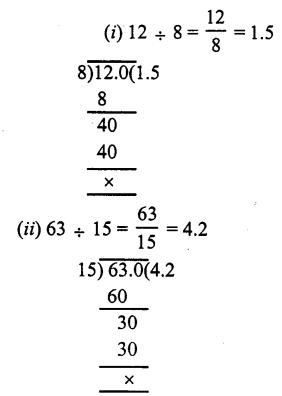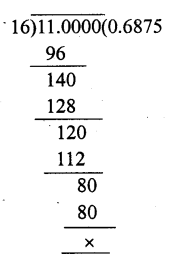Question 5.
Solution: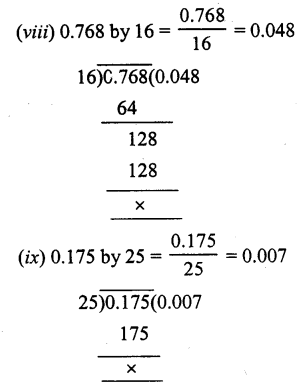Question 6.
Solution: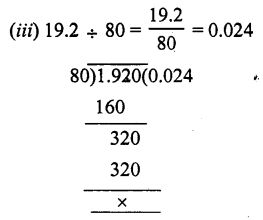Question 7.
Solution: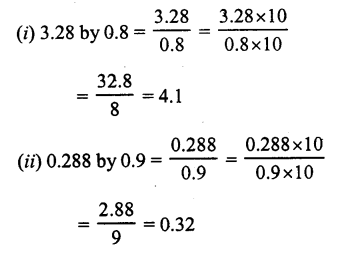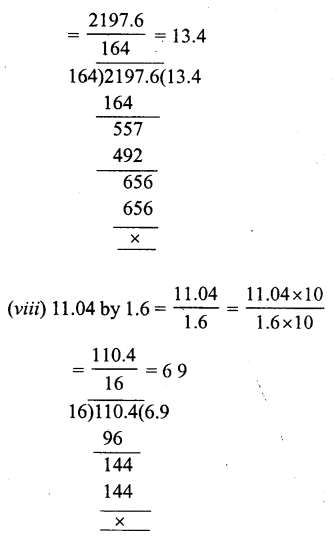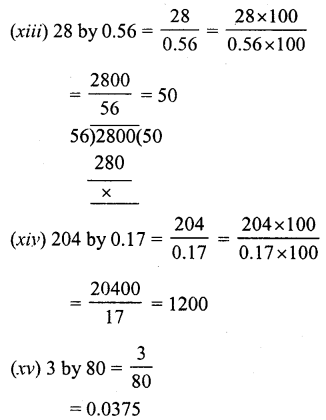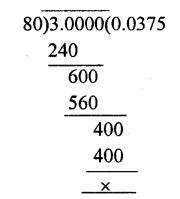Question 8.
Solution:
Cost of 24 chairs = Rs. 9255.60
Cost of one chair = Rs. 9255.6024 = Rs. 385.65

Question 9.
Solution:
Length of cloth for one shirt = 1.8 m
Total length of piece of cloth = 45 m
Number of shirts will be = 45 ÷ 1.8Question 10.
Solution:
A car covers in 2.4 litre = 22.8 km
It will cover in 1 litre = 22.82.4 km = 9.5 km

Question 11.
Solution:
Oil in one tin = 16.5 l
Total oil = 478.5 l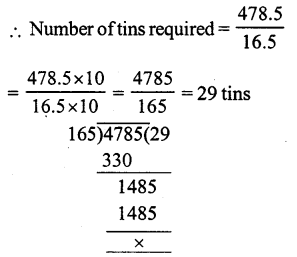Question 12.
Solution:
Weight of 37 bags of sugar=3644.5 kg
Weight of one bag of sugar = 3644.5 ÷ 37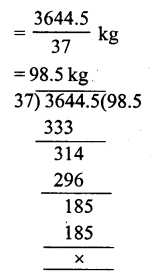Question 13.
Solution:
Capacity of 69 buckets = 586.5 litres
Capacity of 1 bucket = 586.5 ÷ 69Question 14.
Solution:
Number of pieces in 1.15 m = 1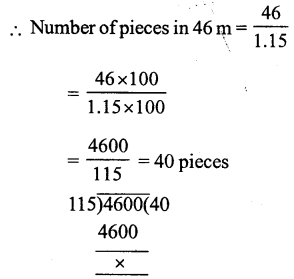Question 15.
Solution:
Total weight of cement = 1792.8 kg
Cement in one bag = 49.8 kg
Number of bags = 1792.8 ÷ 49.8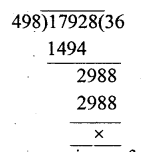Question 16.
Solution:
Total thickness = 1.89 m = 189 cm
Thickness of one piece = 0.3 5 cm
Number of pieces = 189 ÷ 0.35Question 17.
Solution:
Product of two decimals = 261.36
One decimal = 17.6
Second decimal = 261.36 ÷ 17.6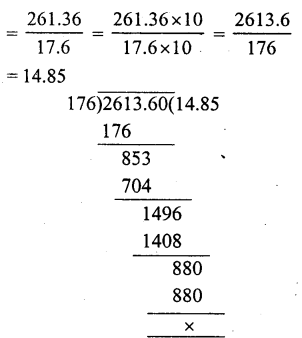All Chapter RS Aggarwal Solutions For Class 7 Maths

—————————————————————————–

All Subject NCERT Exemplar Problems Solutions For Class 7

All Subject NCERT Solutions For Class 7

*************************************************

I think you got complete solutions for this chapter. If You have any queries regarding this chapter, please comment on the below section our subject teacher will answer you. We tried our best to give complete solutions so you got good marks in your exam.

If these solutions have helped you, you can also share rsaggarwalsolutions.in to your friends.